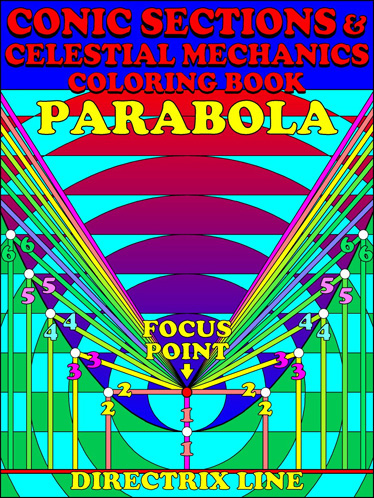My coloring book looks at Dandelin Spheres, as well as other approaches to conic sections. Level: for kids from kindergarten to college. Available at Amazon.com
-
Dandelin Spheres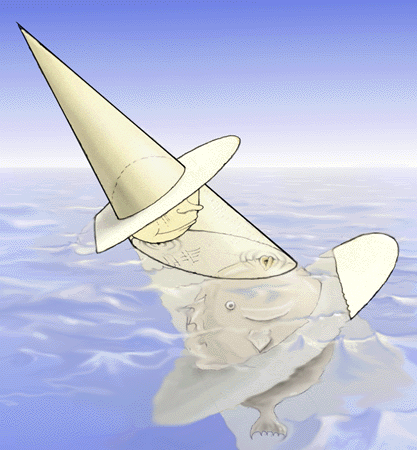A floating ball head wearing a dunce-cap/mosquito-net. Where the ocean surface plane meets the mosquito net is the conic section (here it's an ellipse) Where the ball head touches the water is a focus Where the fish kisses the air is a focus. The ball head's hat brim is a directrix plane as is the fish's belt plane. Where the directrix planes meet the ocean surface are two lines called the directrix lines.
-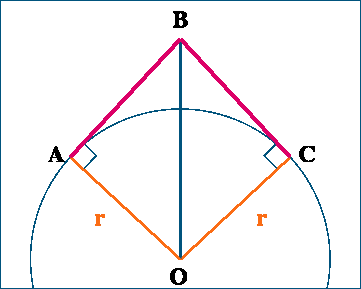Much of Dandelin's demonstrations rests on the fact that two of a sphere's tangent line segments sharing a far end point are equal. AO = r = OC OB = OB Angle OAB and angle OCB are 90 degrees because AB and CB are tangent line segments. AB = sqrt(OB^2 - r^2) = CB because of Pythagarus' theorem.
-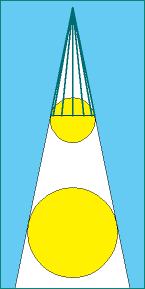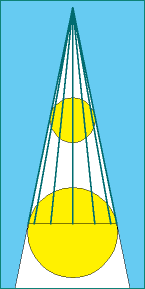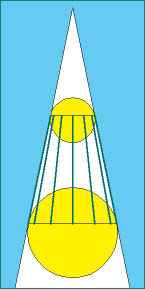Spokes from the hat band are all equal because they are tangent lines sharing a far point. Spokes from the fish's belt are all equal length for the same reason. Take away hat spokes from belt spokes and you're left with  lamp shade spokes which are all equal.
-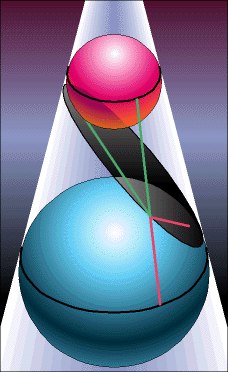Green lines from hat band to ellipse and from focus to the same point are tangent line segments sharing a far point. Same for the orange lines. Green plus orange line segments make a lamp shade spoke. Tracing the ellipse we see Distance to 1st focus + Distance to 2nd focus = lamp shade spoke.
-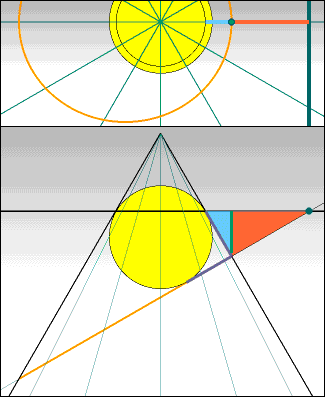Drop a hinge line straight off hat brim to a point on ellipse. Hinge line is a leg of both blue and red right triangles. It forms a constant angle with the cone wall (on which the blue hypotenuse rests) and a constant angle with the cutting plane (on which the red hypotenuse rests). Blue hypotenuse is the point's distance to focus (can you see why?) Red hypotenuse is the point's distance to directrix line. Since the triangles remain the same shape and share a leg the ratio of blue hypotenuse to the red hypotenuse remains constant. This constant is called the ellipse's eccentricity. - An ellipse's eccentricity is between 0 and 1. A circle's eccentricity is called zero although it's directrix line is not defined.
-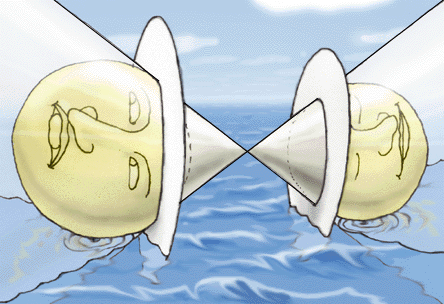Some mischieveous lout has glued the ballhead brothers together! As before, where the mosquito net meets the ocean is the conic section (This time it's a hyperbola). Where the ballheads touch the ocean are the foci, the hatbrims are the directrix planes and the water line on the hat brims are the directrix lines.
-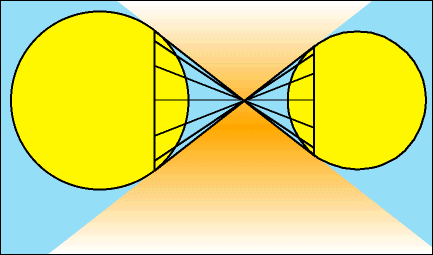As before all the little ball's hatspokes are equal. Ditto for the big ball's hat spokes. The big and and little hat spokes together make equal hourglass spokes.
-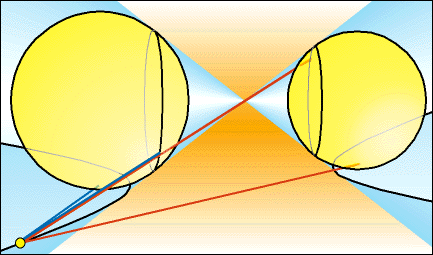Let a blue line show a point's distance to the near focus and a red line it's distance to the far focus. Both the red and blue line have twins that start from the hat brims. From the hatbrim lines you can see that Distance to 1st focus - Distance to 2nd focus = hourglass spoke.
-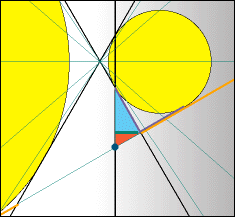As with the ellipse, extend a perpendicular hinge from the hat brim to a point on the hyperbola. The hinge is a shared leg of 2 right triangles. The blue hypotenuse is distance to focus and the red hypotenuse is distance to directrix line. As with the ellipse, the ratio of the blue to the red hypotenuse is constant and this constant is the hyperbola's eccentricity. A Hyperbola's eccentricity is always greater than 1.
-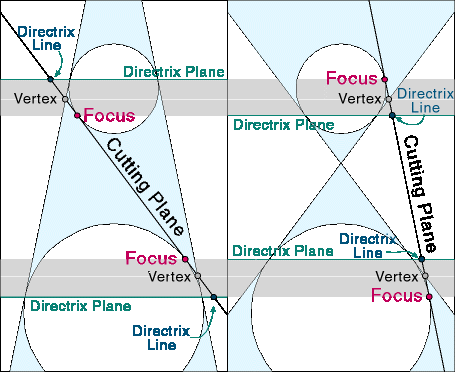A pair of Dandelin spheres define two tangent cones: the thin cone with its apex on the far side of the little sphere, and the fat cone with its apex between the spheres. They also define two sets of cutting planes which are tangent planes to either the thin or fat cone. Planes tangent to the fat cone will cut the thin cone forming ellipses of a specific eccentricity. Planes tangent to the thin cone will cut the fat cone forming hyperbolas of a specific eccentricity. Ellipses' eccentricity = 1/hyperbolas'  eccentricity.
-Tilting so the top edge of the mosquito net is parrallel with the ocean, the ball head looks up and down to see no brother or fish is around. This conic section has only one focus. It is the parabola.
-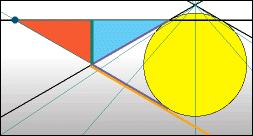Dropping the hinge from the hat brim we can see the blue hypotenuse is the same as the red hypotenuse. Distance to directrix = Distance to focus and the parabola's eccentricity is exactly 1.

Some conic moires I've done:
Hyperbola/ellipse moires
Parabola moire
Conics of varying eccentricity moire